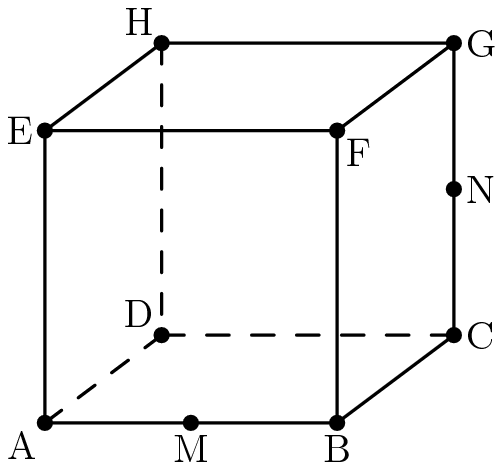# Volume of a Part of a CubeCube $ABCDEFGH$, labeled as shown above, has edge length $1$ and is cut by a plane passing through vertex $D$ and the midpoints $M$ and $N$ of $\overline{AB}$ and $\overline{CG}$ respectively. The plane divides the cube into two solids. The volume of the larger of the two solids can be written in the form $\frac{p}{q}$, where $p$ and $q$ are relatively prime positive integers. Find $p+q$.

×